# Prime Factorization

##By Mathademics

Prime Factorization# Prime Factorization

##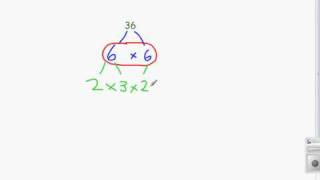By TeacherTube Math

WEBSITE: http://www.teachertube.com Finding the prime factorization of a number using factor trees# Example 1: Factoring trinomials with a common factor | Algebra II | Khan Academy

##By Khan Academy

u12_l2_t1_we2 Factoring trinomials with a common factor. All Khan Academy content is available for free at www.khanacademy.org# Distributive Property and Factoring

##By Dr.Steeve Warner

Distributive Property and Factoring# The Factor Theorem : ExamSolutions

##By ExamSolutions

The Factor Theorem : ExamSolutions# Finding LCM by Listing Factors

##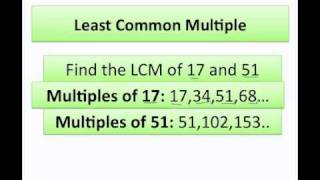By Vanessa Graulich

Learn to find LCM of numbers by listing their factors.# Factoring polynomials

##By MathPlanetVideos

Factor 2x2-4xy+2y2=2(x2-2xy+y2)=2(x-y) 2# The Factor Theorem

##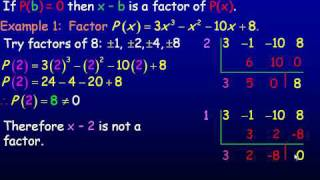By AlRichards314

YouTube presents The Factor Theorem, an educational video resource on math.# Factoring Polynomials

##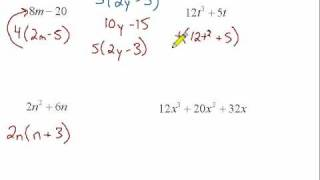By Marty Brandl

This video looks at factoring simple binomials and trinomials. It includes four examples.# Finding Factor Pairs

##By Skubes ed

Find all factor pairs for a whole number in the range 1–100.# Solving quadratics by factoring 2

##By Khan Academy

Sal solves the equation s^2-2s-35=0 by factoring the expression on the left as (s+5)(s-7) and finding the s-values that make each factor equal to zero.# Solving quadratics by factoring 2

##By Khan Academy

Sal solves the equation s^2-2s-35=0 by factoring the expression on the left as (s+5)(s-7) and finding the s-values that make each factor equal to zero.# Solving quadratics by factoring

##By Khan Academy

Sal solves the equation s^2-2s-35=0 by factoring the expression on the left as (s+5)(s-7) and finding the s-values that make each factor equal to zero.# How to Factor Polynomials by Grouping

##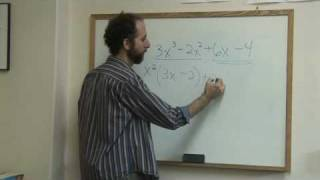By eHow

In math, factoring by grouping means that the greatest common factor needs to be found. In this video learn how to factor four different terms through grouping with help from a math author and teacher. As narrator explains there is text of what he says shown on the bottom of the screen.# Remainder and factor theorems

##By MathPlanetVideos

Is (x-1) a factor of f(x)=x4-2x2+1?# Finding factors of a number | Factors and multiples | Pre-Algebra | Khan Academy

##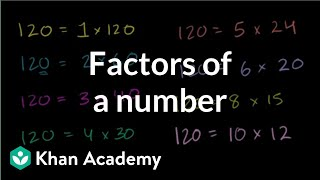By Khan Academy

To find the factors of a number means to find all the whole numbers that the number in question is divisible by. Can you help us find the factors in this example?# Factoring Fun (4.OA.B.4)

##By Madison`s Math

fourth graders are expected to find all factor pairs for the numbers 1 through 100.# Factor differences of squares

##By Khan Academy

Sal factors 45x^2-125 as 5(3x+5)(3x-5).# Factor polynomials with quadratic forms

##By Khan Academy

Sal factors 5rs+25r-3s-15 as (s+5)(5r-3).# Understand Division as an Unknown Factor Problem

##By Hoodamath tutorials

division problems with unknown factors